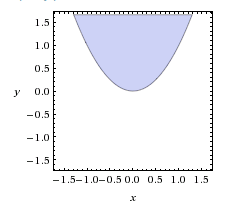# A Hacky Alternative to Image Identification Using Grids

Posted on June, 24 2015

Since my last post about R3CI, a whole lot of progress has been done on the project. The server side is up to par, implementing modular protocol type handlers. The client side is up to the bare minimum, supplying the baseline functions for the final test.

As expected of one critical component of R3CI, classifying all of the robots could not be done within the time-span given to us. An unfortunate statement to make indeed, but this allows me to test how robots behave under a 'blindfold' per-se. The solution to this problem is to rely on the coordinate grid alone, and identifying robots based solely on the relative coordinates of robots in the grid. Of course, this is not ideal due to imperfections within accurately updating the robots coordinates. For now, we will assume the coordinate grid is accurate.

The overview of the idea is to 'simulate' the area of vision the image would produce, using only the relative x,y coordinates of each robot. This can be implemented in many ways, but I chose to use a quadratic function inequality to render a robot 'in view' and 'out of view'. This may seem hacky, but- well, it's because it is.

``````y > x^2 + x_off*x + y_off
``````

And it's output where `x_off` and `y_off` both equal 0 isOn the coordinate grid, the robot trying to see what is in front of itself exists at 0,0 (This is why `x_off` and `y_off` are set to 0 in the example). Any coordinate in the light blue shaded region is where a robot will be 'seen'. This is great and all, but it has a shortfall- The angle of the robot trying to 'see' must be at 90 degrees.

The step that must be done before you check whether a robot is in sight or not is to rotate the entire coordinate plane thus that the robot's angle is 90 degrees relative to every other robot. This can be done with two simple functions for x and y.

``````f(x) =  cos(angle)x + sin(angle)y

f(y) = -sin(angle)x + sin(angle)y
``````

What this produces is the coordinates of x,y translated by the angle specified. Translating the coordinate plane thus that the robot's angle is 90 degrees, and feeding in the quadratic equation should yield the desired results.

Overall, this solution is a good way to roughly simulate what using image identification can do with much less processing power. For this to be implemented in the future though, keeping the coordinate grid accurate is a must.